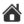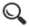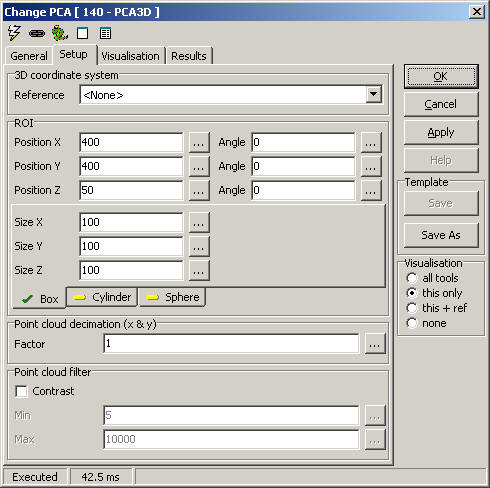Up | CircleFinder2D - 165 | EasyOCR - 78 | PolygonMatch2 - 84 | GCPS -110 | HeightMap3D - 111 | ImageExtrema - 129 | PCA3D - 140 | CameraPose3D - 148 | MonoPose3D - 149 | ImagePicker3D - 145 | ExternalCoordinates3D - 157 | MaMa3D - 152 | CircleSegmentor - 153 | PolygonMatch3 - 160PCA3D - 140

The PCA (Principal Component Analysis) tool searches a point cloud within an ROI, and returns the principal directions and corresponding eigenvalues (roughly related to the square of the extent in that direction). The major (X) axis of the returned reference system will be aligned with where the point cloud has the largest spread.

Setup

3D coordinate system

• Reference - select a 3D reference for position and results

ROI

Point cloud decimation (x & y)

• Factor - keep only every nth sample in x and y directions

Point cloud filter

• Contrast - element 4 in a 5-element point cloud; set min and max allowable valueVisualisation
 ROI The ROI cube/cylinder segment/sphere Points All points within the ROI Eigenvectors The lengths are visualized as the square root of the eigenvalues

Results

 Center.x/.y/.z The mean of all points Point count Number of points within the ROI Eigenvector1.x/.y/.z Normalized eigenvector 1 (major axis) Eigenvector2.x/.y/.z Normalized eigenvector 2 Eigenvector3.x/.y/.z Normalized eigenvector 3 (minor axis) Eigenvalue1 Describes the spread in this direction Eigenvalue2 Describes the spread in this direction Eigenvalue3 Describes the spread in this direction

ExecuteCmd support